# Universal algebra

An algebraic system with an empty set of relations. A universal algebra is frequently simply called an algebra. For universal algebras the homomorphism theorem holds: Ifis a homomorphism from one universal algebraonto another algebraandis the kernel congruence of, thenis isomorphic to the quotient algebra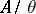. Every universal algebra may be decomposed into a subdirect product of subdirectly-irreducible universal algebras.

If to the basic operations of an algebraone adjoins all derived operations, one obtains a universal algebraof larger signature. The equation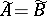is possible even for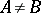, which leads to the notion of rational equivalence of universal algebras (cf. Variety of universal algebras).

With every universal algebrathere are associated related structures: the monoid of all endomorphisms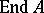, the group of all automorphisms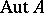, the lattice of all subalgebras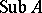, and the lattice of all congruences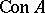. For any groupand any algebraic lattices (cf. Algebraic lattice)andthere exists a universal algebrasuch that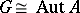,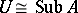and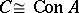(cf. ). However, ifis replaced by, the corresponding result does not hold. This kind of problem is called an abstract realization problem. An example of a solution of a concrete realization problem is: A system of subsetsof a setcoincides withfor some universal algebra with carrierif and only ifis closed under directed unions and arbitrary intersections . The abstract, as well as the concrete, realization problem can be solved for given classes of universal algebras. Universal algebras with some restriction on the related structures have been studied. E.g., one studies universal algebras with distributive or modular congruence lattices, with-element congruence lattices (congruence-simple universal algebras), with-element or-element lattices of subalgebras (simple universal algebras), with a commutative monoid of endomorphisms, with a-element group of automorphisms (rigid universal algebras), etc. A universal algebra with commuting congruences is isomorphic to the direct product of a finite number of congruence-simple algebras if and only if its congruence lattice satisfies the maximum condition, and the least upper bound of its minimal congruences is equal to the largest congruence. Universal algebras with a distributive congruence lattice and with permutable congruences (arithmetic universal algebras) admit a representation as global sections of a suitable sheaf. A study has been made to what extent a universal algebra is determined by some of its related structures. However, the majority of results of this kind concern concrete classes of universal algebras (, ).

A universal algebra is called functionally complete if every operation on its carrier belongs to the clone generated by its basic operations and by the constants. If one excludes constants, one obtains primal (or strictly functional complete) universal algebras. If all congruence-preserving operations lie in the above-mentioned clone, one obtains an affine complete universal algebra. Every functionally complete universal algebra is finite. Therefore, the requirement of finiteness is often included in the definition of these classes of universal algebras (cf. , , ).

The study of universal algebras was begun in the 1930's and 1940's, when the basic definitions were formulated, varieties of universal algebras were characterized and the subdirect decomposition theorem was proved (cf. , ). The earlier history of the theory of universal algebras goes back to the 19th century. The active study in this domain began in the USSR in the early 1950's (A.G. Kurosh, A.I. Mal'tsev, and their pupils). The use of methods of mathematical logic led to the consideration of algebraic systems.

The expression "universal algebra" is often used in the sense of "the theory of universal algebras" .